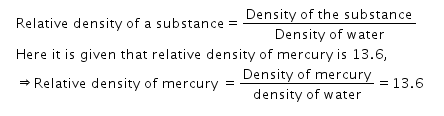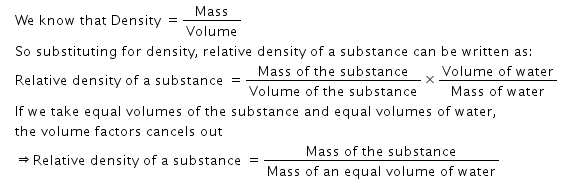# Relative density of mercury is 13.6. What does this statement mean?

Relative density of mercury is 13.6. What does this statement mean?

Relative density of a substance is the ratio of its density to that of water.ie.So relative density of mercury is 13.6 means that density of mercury is 13.6 times the density of water.
Or in other terms:Hence we can say that relative density of mercury is 13.6 means that mercury is 13.6 times as heavy as an equal volume of water.